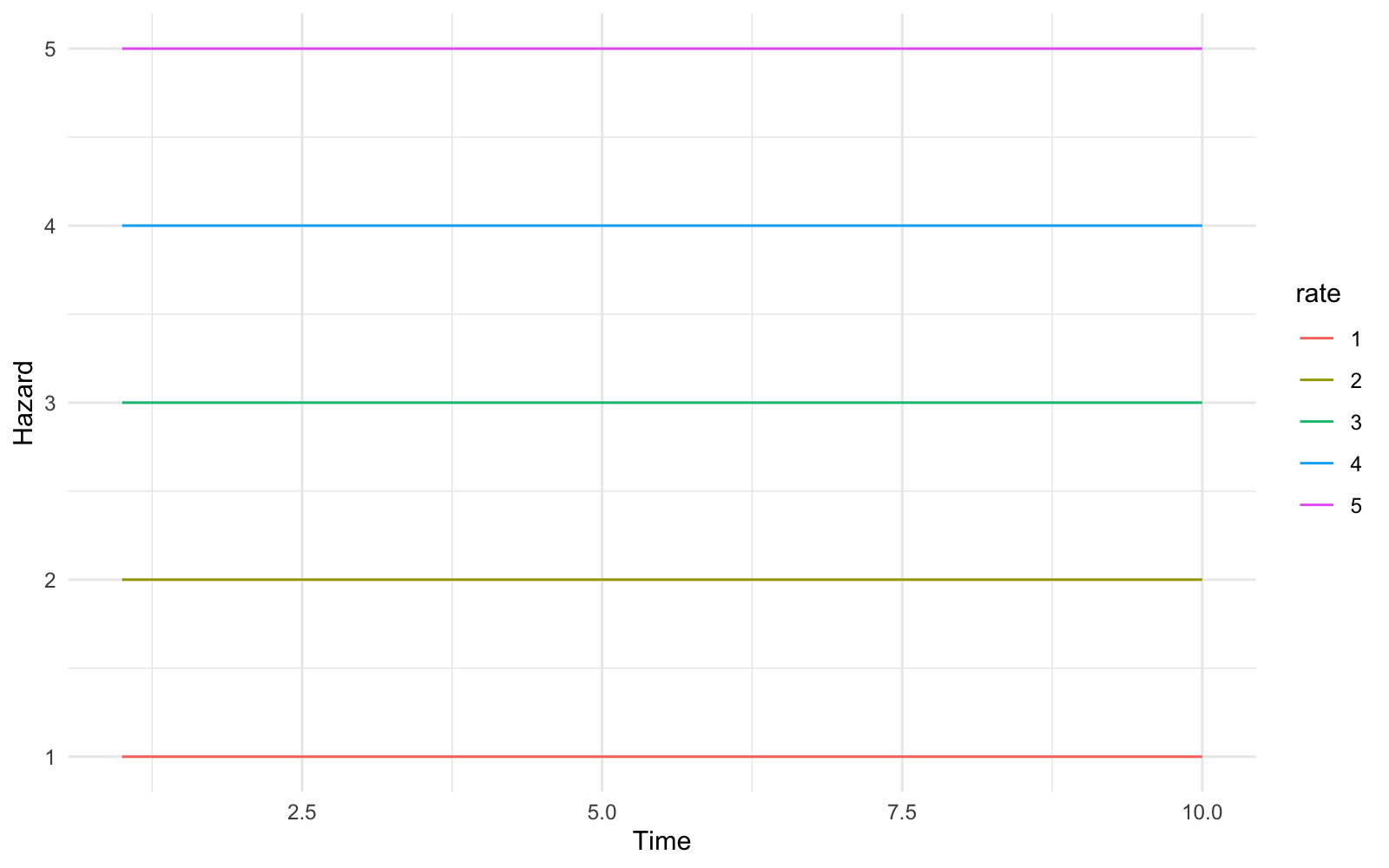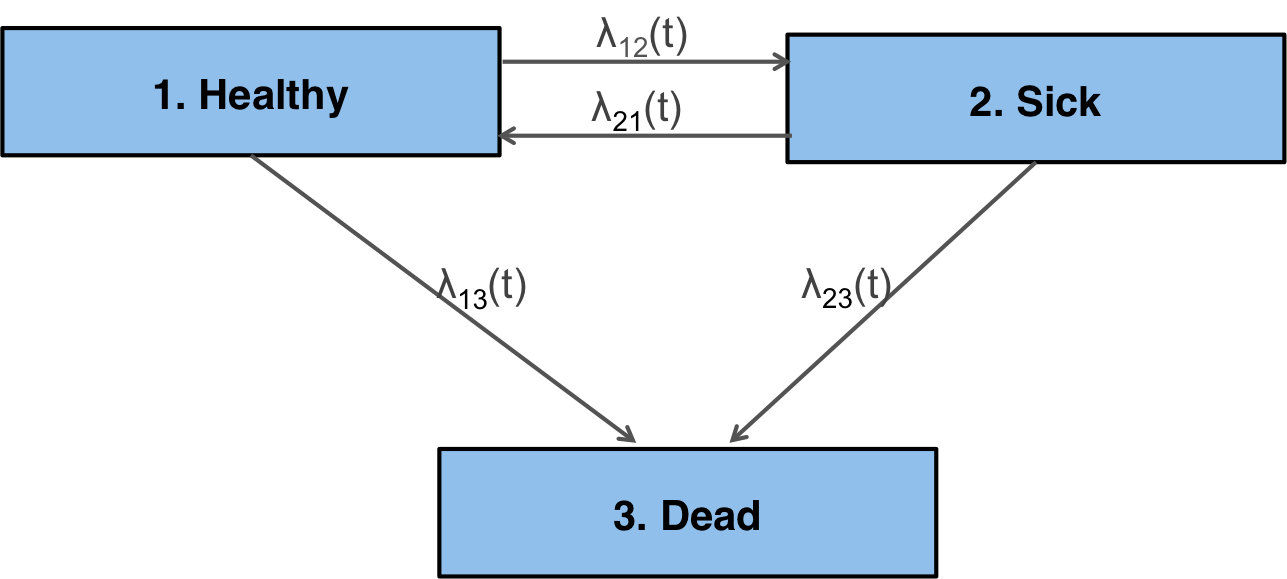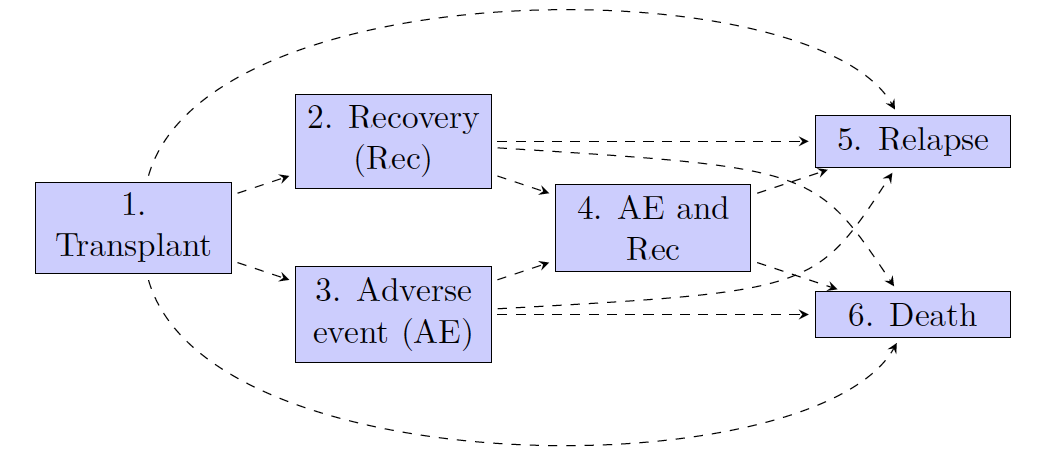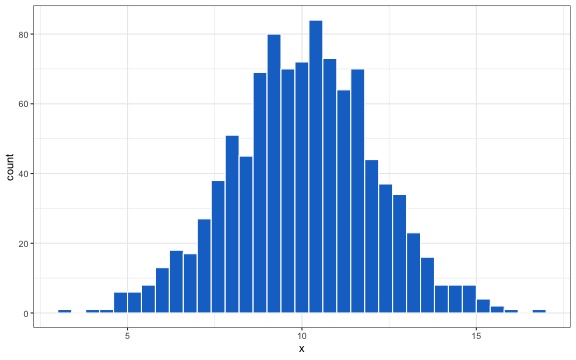# Blog Archives

## Parametric survival modeling

June 17, 2019
ByIntroduction Survival distributions Shapes of hazard functions Exponential distribution Weibull distribution (AFT) Weibull distribution (PH) Gompertz distribution Gamma distribution Lognormal distribution Log-logistic distribution ...

## Cost-effectiveness analysis with multi-state and partitioned survival models: hesim 0.2.0

April 2, 2019
ByOverview An example CTSTM Decision problem and model setup Parameterization Simulation Constructing the economic model ...

## Simulating Multi-state Models with R

December 31, 2018
ByIntroduction An example 6-state model Estimation Data Fitting Simulation mstate hesim Comparison ...

## Simulating Multi-state Models with R

December 31, 2018
ByIntroduction An example 6-state model Estimation Data Fitting Simulation mstate hesim Comparison ...

## Probabilistic Sensitivity Analysis with R

February 9, 2018
ByOverview Normal distribution Multivariate normal distribution Beta distribution Dirichlet distribution Gamma and lognormal distributions Uniform distribution Performing a PSA Overview Decisions to adopt or reimburse health technologies are often guided by cost-effectiveness analyses. The outcomes needed to conduct cost-effectiveness analyses are simulated using decision-analytic models, which combine information from multiple sources and extrapolate outcomes...

## Probabilistic Sensitivity Analysis with R

February 9, 2018
ByOverview Normal distribution Multivariate normal distribution Beta distribution Dirichlet distribution Gamma and lognormal distributions Uniform distribution Performing a PSA Overview Decisions to adopt or reimburse health technologies are often guided by cost-effectiveness analyses. The outcomes needed to conduct cost-effectiveness analyses are simulated using decision-analytic models, which combine information from multiple sources and extrapolate outcomes...